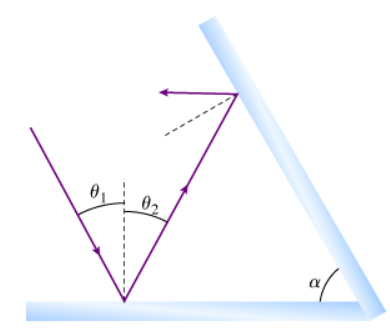# Problem: If the light strikes the first mirror at an angle θ1, what is the reflected angle θ2?

###### FREE Expert Solution

For plane mirrors, the angle of reflection is the same as the angle of incidence.

93% (207 ratings)###### Problem Details

If the light strikes the first mirror at an angle θ1, what is the reflected angle θ2?